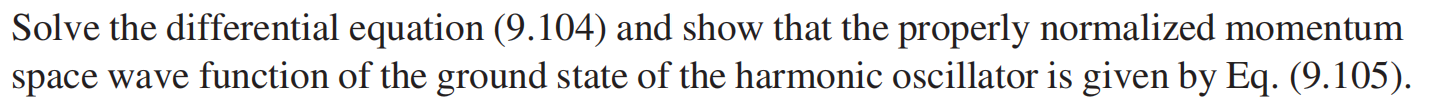# Question Solve the differential equation (9.104) and show that the properly normalized momentum space wave function of the ground state of the harmonic oscillator is given by Eq. (9.105).B3SM7O The Asker · Mechanical Engineering
Solve the differential equation (9.104) and show that the properly normalized momentum space wave function of the ground state of the harmonic oscillator is given by Eq. (9.105).Transcribed Image Text: Solve the differential equation (9.104) and show that the properly normalized momentum space wave function of the ground state of the harmonic oscillator is given by Eq. (9.105).
More
Transcribed Image Text: Solve the differential equation (9.104) and show that the properly normalized momentum space wave function of the ground state of the harmonic oscillator is given by Eq. (9.105).